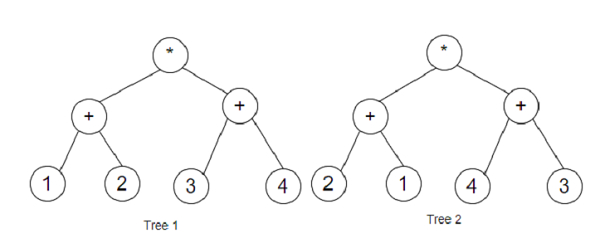# Program to find out if two expression trees are equivalent using Python

Suppose, we are provided two expression trees. We have to write a program that checks the two expression trees and determines if the expression trees generate similar values. The two expression trees are provided to us in an in-order manner and we return a True value if they match, or else we return a False value.

So, if the input is likethen the output will be True.

The two expression trees evaluate to the same value.

To solve this, we will follow these steps:

• Define a function dfs() . This will take node, dic

• if node is empty, then

• return

• if left of node and right of node is not empty, then

• dic[value of node] := dic[value of node] + 1

• dfs(left of node, dic)

• dfs(right of node, dic)

• dic1 := a new map containing integer values

• dic2 := a new map containing integer values

• dfs(root1, dic1)

• dfs(root2, dic2)

• return True if dic1 is same as dic2.

## Example

Live Demo

import collections
class TreeNode:
def __init__(self, val=0, left=None, right=None):
self.val = val
self.left = left
self.right = right
def insert(temp,data):
que = []
que.append(temp)
while (len(que)):
temp = que
que.pop(0)
if (not temp.left):
if data is not None:
temp.left = TreeNode(data)
else:
temp.left = TreeNode(0)
break
else:
que.append(temp.left)
if (not temp.right):
if data is not None:
temp.right = TreeNode(data)
else:
temp.right = TreeNode(0)
break
else:
que.append(temp.right)
def make_tree(elements):
Tree = TreeNode(elements)
for element in elements[1:]:
insert(Tree, element)
return Tree
def solve(root1, root2):
dic1 = collections.defaultdict(int)
dic2 = collections.defaultdict(int)
def dfs(node, dic):
if not node:
return
if not node.left and not node.right:
dic[node.val] += 1
dfs(node.left, dic)
dfs(node.right, dic)
dfs(root1, dic1)
dfs(root2, dic2)
return dic1 == dic2
root1 = make_tree([1, '+', 2, '*', 3, '+', 4 ])
root2 = make_tree([2, '+', 1, '*', 4, '+', 3])
print(solve(root1, root2))

## Input

root1 = make_tree([1, '+', 2, '*', 3, '+', 4 ])
root2 = make_tree([2, '+', 1, '*', 4, '+', 3])

## Output

True